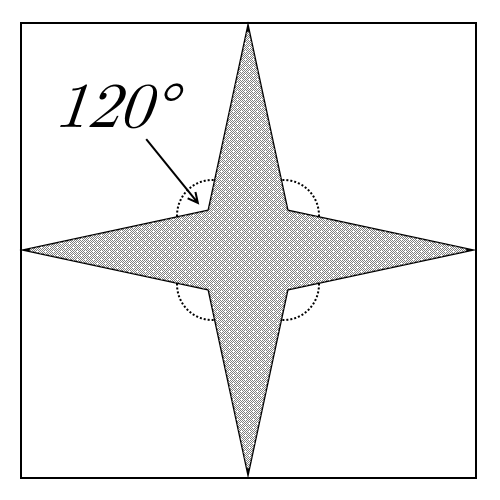# Star Stumper

Geometry Level 4A square $ABCD$ of side length $2$ has a 4-pointed star inscribed in it - its points touch the midpoints of each side of the square, and it has lines of symmetry $AC$ and $BD$.

Given that the angle between the points is $120^\circ$, the area of the shaded region can be written as $\dfrac { a-b\sqrt { 3 } }{ c }$ where $a$, $b$ and $c$ are coprime. Find $a+b+c$.

×

Problem Loading...

Note Loading...

Set Loading...